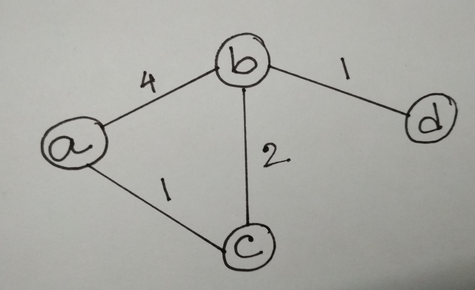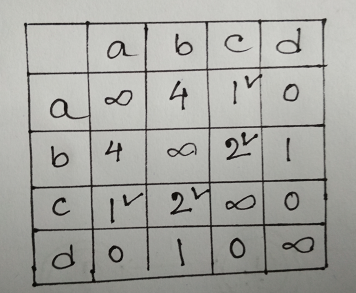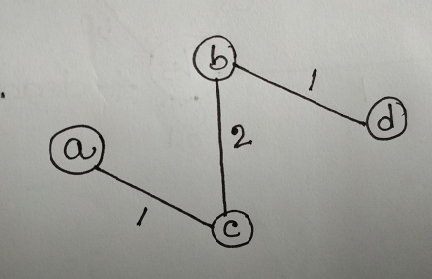# Question & Answer: Let G = (V, E, c), where E = {(a,b,4),(a,c,1),(c,b,2),(b,d,1). Draw the graph and…..

Let G = (V, E, c), where E = {(a,b,4),(a,c,1),(c,b,2),(b,d,1). Draw the graph and construct the distance traversal tree rooted at a.

Don't use plagiarized sources. Get Your Custom Essay on
Question & Answer: Let G = (V, E, c), where E = {(a,b,4),(a,c,1),(c,b,2),(b,d,1). Draw the graph and…..
GET AN ESSAY WRITTEN FOR YOU FROM AS LOW AS \$13/PAGE

Solution:

Graph:

Following is an undirected weighted graph. The weight of the edge between a and b is 4, the edge between a and c is 1, the edge between b and c is 2 and the edge between b and d is 1.Distance traversal tree:

The tree rooted at node a is created such that :

• number of edges = number of vertices – 1(which is the characteristic of a tree) = 4 – 1 = 3.
• and the edges are chosen such that the total distance traversed from root a to d is minimum.

To construct the tree the rules written below are to be followed:

• Construct an adjacency matrix. An edge between a vertex v to v is marked as  (infinity) to denote prevention of self loop in the tree. An edge between two vertices v and u are marked as 0 if there is no edge available between them.
• Start from the root node s. Find the minimum edge e corresponding to the row of the root node. Mark the edge s->v and go to the row corresponding to v. Mark e in that row and find the minimum edge in that row ( except edge e).
• Repeat the previous step until an edge makes a circuit in the tree.

​​